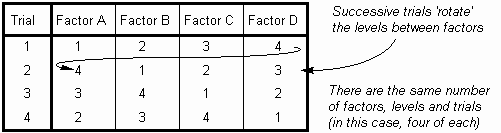The Psychology of Quality and More

Design of Experiments (DOE): Practical variations

The Quality Toolbook > Design of Experiments (DOE) > Practical variations

Practical variations

• Use a Matrix Diagram to select a subset of trials from the full factorial, as illustrated. Rows and columns are allocated to factors and their levels such that each square represents a unique combination. Squares are then shaded to represent actual trials to use. A separate key may be used to describe the factors and levels.
• Results may be recorded on the same matrix, and summed in matching boxes opposite the factor/level headings.Fig. 1. Using a Matrix Diagram

• A Latin Square is a simple method of selecting a small set of trials in a situation where there are several factors and levels. The square simply ensures that each level occurs once for each factor by rotating the levels around the factors for each successive trial, as illustrated. If the resulting pattern may affect the result, the trials may be performed in random order.
• Latin squares have a fairly significant constraint in that there must be the same number of factors, levels and trials. This may be achieved by selecting the number of levels to match the number of factors.Fig. 2. Latin Square

<-- Previous | Next -->

You can buy books here

And the big
paperback book